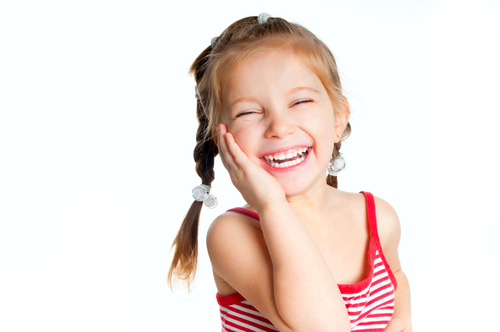# Practise Solving Multiplication and Division Word Problems

In this worksheet, students will need to solve a variety of multiplication and division word problemsKey stage:  KS 1

Curriculum topic:   Number: Multiplication and Division

Curriculum subtopic:   Solve Problems Using Multiplication/Division

Difficulty level:#### Worksheet Overview

In this activity, we will be solving multiplication and division word problems.

Let's have a look at the steps needed to tackle word problems:

2. Is this a division or multiplication word problem?

3. What is the number sentence for this word problem?

4. Now, solve the problem by working out the answer.

5. Check, read the question again, and check the answer makes sense.

Here are a few things to remember:

Multiply means 'lots of' or 'groups of' and the answer will always be bigger.

Divide means 'shared by' and the answer will always be smaller.

Now, let's have a look at some examples.

Example 1There are 8 cats.

Each cat has 2 eyes.

How many eyes do the cats have altogether?

Let's go through the steps to work this out:

2. This is a multiplication sentence as there are 8 'groups of' 2 eyes and we need to work out how many altogether.

3. 8 'groups of' 2 would be written: 8 x 2 =

4. 8 x 2 = 16

5. There are 16 eyes altogether - yes it works.

Example 2There are 20 fish.

Each fishbowl holds 5 fish.

How many fish bowls are needed for the 20 fish?

Let's go through the steps to work this out:

2. This is a division sentence as we are starting with 20 fish and sharing the fish into groups of 5.

3. 20 shared by 5 would be written: 20 ÷ 5 =

4. Remember, this means, how many 5s are in 20? 20 ÷ 5 = 4

5. 4 fish bowls will be needed - yes this works.

Let's have a go at some multiplication and division word problems!### What is EdPlace?

We're your National Curriculum aligned online education content provider helping each child succeed in English, maths and science from year 1 to GCSE. With an EdPlace account you’ll be able to track and measure progress, helping each child achieve their best. We build confidence and attainment by personalising each child’s learning at a level that suits them.

Get started